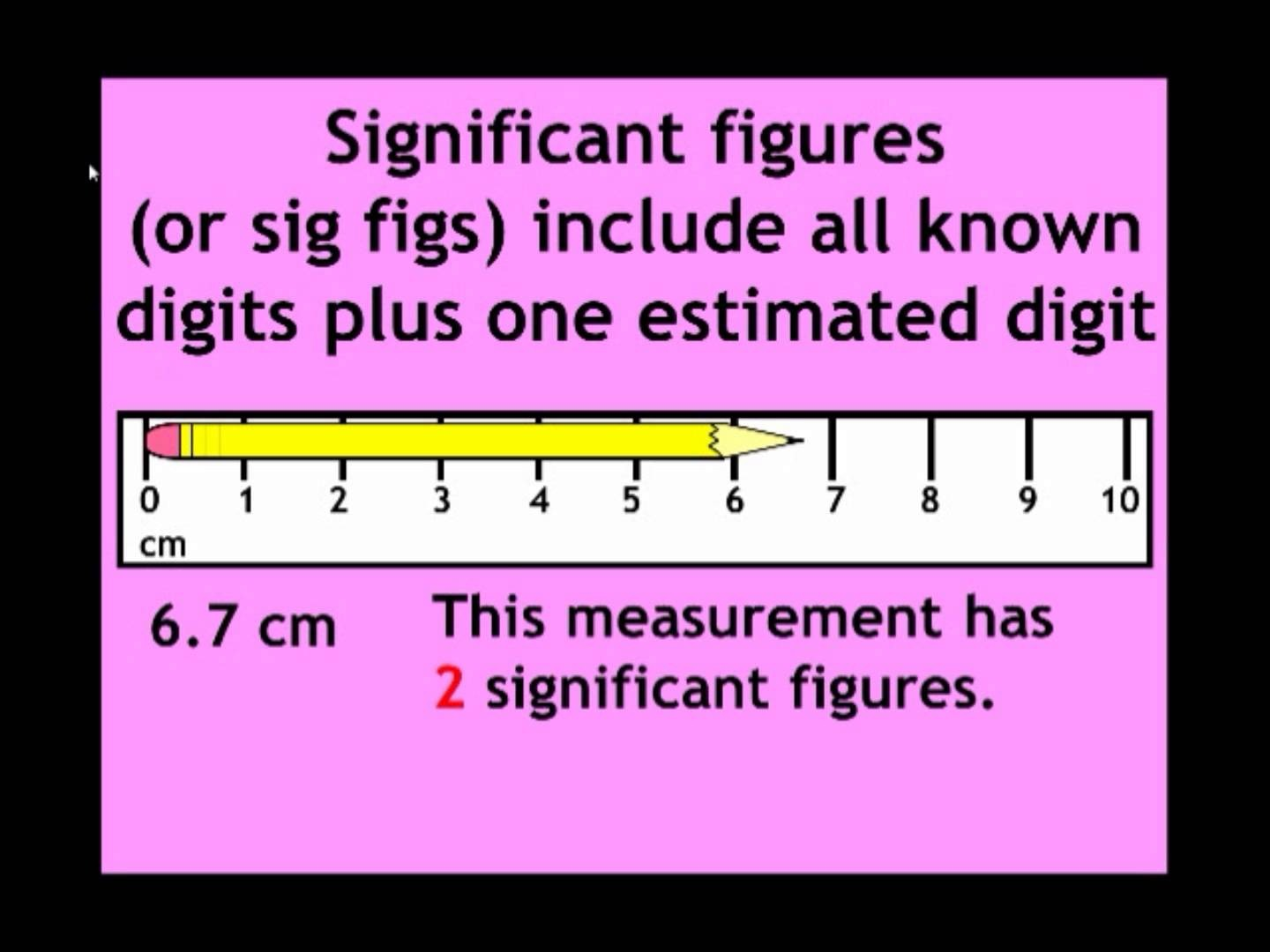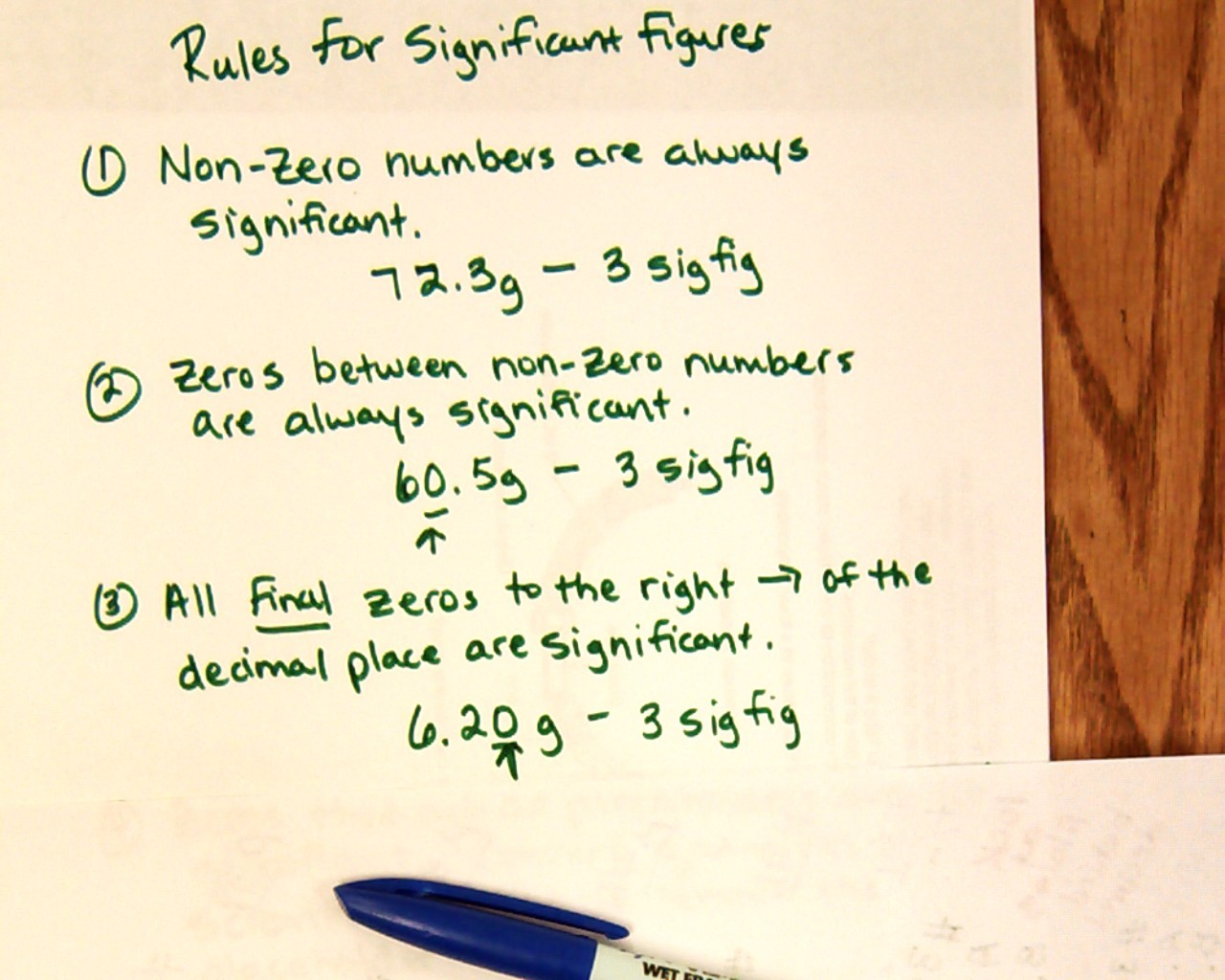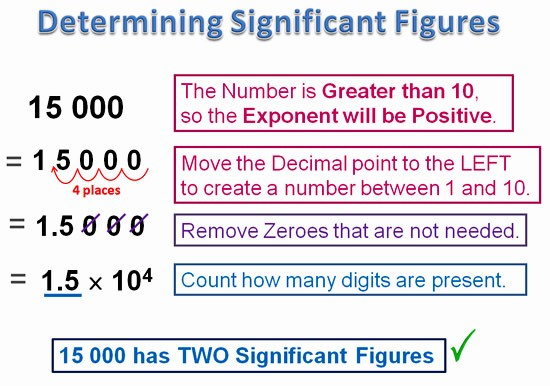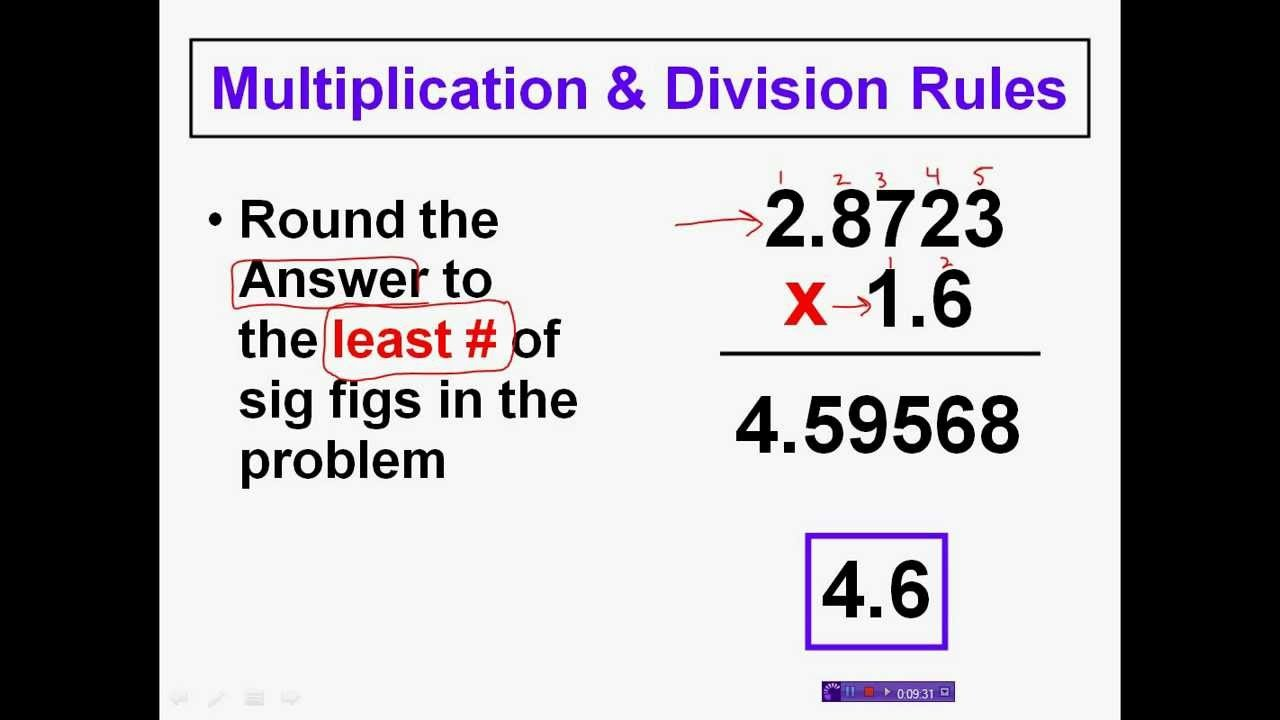×#### Thank you for registering.

One of our academic counsellors will contact you within 1 working day.

Click to Chat

1800-1023-196

+91-120-4616500

CART 0

• 0

MY CART (5)

Use Coupon: CART20 and get 20% off on all online Study Material

ITEM
DETAILS
MRP
DISCOUNT
FINAL PRICE
Total Price: Rs.

There are no items in this cart.
Continue Shopping• Kinematics & Rotational Motion
• OFFERED PRICE: Rs. 636
• View Details

```Significant Figures

Table of Content

Introduction to Significant Figures

Accuracy

Precision

Significant Figures

How to determine Significant Figures?

Using scientific notation to determine significant digits

Rounding off Uncertain Digits

Rules for Arithmetic Operation with significant number

Multiplication and Division

Introduction to Significant Figures

Every measurement comes with an error. Measurement depends on two factors, namely precision, and accuracy. These two factors help in determining the existence of physical quantities and help in comparing and measuring them.Accuracy

Accuracy is defined as the closeness of measured value to a standard value. Suppose you weigh a box and noted 3.1 kg but its known value is 9 kg, then your measurement is not accurate.

Precision

Precision is defined as the closeness between two or more measured values to each other. Suppose you weigh the same box five times and get close results like 3.1, 3.2, 3.22, 3.4, and 3.0 then your measurements are precise.

Remember: Accuracy and Precision are two independent terms. You can be very accurate but non-precise, or vice-versa.

Measurements of units revolve around accuracy and precision. The result of a measurement should be precise. The measurement of a physical quantity involves two digits. The digits are of two types; Reliable and Uncertain.

Example

Length of a rope is 1.23 m, then 1 is a reliable digit, 2 and 3 are uncertain digits.

Significant Figures

A Significant Figure or number is a number or digit, which tells how accurate and precise our measurement is. Measuring anything requires a specific type of measuring instrument. Significant Figures make measurements easy and state them precisely.

How to determine Significant Figures?The rules for determining significant figures can be understood from the following example:

Consider a number 10.46 cm, we find digits ‘1', ‘0’ and ‘4’ to be certain and ‘6’ is uncertain. All the certain and uncertain digits are called significant digits, therefore the number has 4 significant digits.

A choice of change of different units doesn’t change the number of significant digits in a measurement. Suppose the length of a string is 5.209 cm, it has four significant digits namely 5, 2, 0 and 9. What if the number is rewritten in a different format? How we will determine the number of significant digits in 0.05209 meters or 52.09 mm or 52090 µm.

The number of significant digit remains same in all the above numbers regardless of the unit of the number. Note down following rules while determining number of significant figures

All the non-zero digits are counted as significant

All the zeroes between two non-zero digits will be counted as significant regardless of position of decimal point or unit

Example: 200.3 has four significant digits

In the number less than one, the zeroes  before decimal and after decimal are counted as insignificant

Example: 0.002456 has four significant digits, and the starting three zeroes are non-significant

The terminal or trailing zeroes in a number without a decimal point are termed as non-significant

Example: 125 m = 12500cm= 125000 mm has three significant figures as the trailing or terminal zeroes are insignificant

The terminal zero in a number with a decimal point is considered as significant.

Example: 4.700 and 0.06890 have four significant figures in each.

Zeroes before a decimal point are counted as insignificant, but zeroes after decimal point are counted as significant

Example: 0.3240 has four significant figures (3, 2, 4 and the last 0)

The multiplying/ dividing factors which always yield a single answer, have infinite number of significant figures

Perimeter of circle is 2πr, factor 2 can be written as 2.0, 2.00 or 2.000 and can have infinite number of significant figuresUsing scientific notation to determine significant digitsTo determine Significant Figures easily without getting confused with trailing concept, we write the number in scientific notation form. Scientific notation means expressing a number in power of 10, that is in the form of a × 10b where a is any number and b is any positive or negative power of 10. We round off a to 1 if a is less than or equal to 5, and to 10 if a is between 5 and 10. b is also called Order of Magnitude.

Example:

The diameter of the earth is 1.28 × 107, has an order of magnitude 7.

The diameter of a hydrogen atom is 1.06 × 10-10 has an order of magnitude -10.

Note: Whenever we express a number in scientific notation, we always write decimal after the first digit.

The power of 10 is insignificant and all the above examples have only 3 significant figures. Scientific notation avoids the trailing zero confusion, as it simply helps in determining the significant figures in the number.

Examples:

1.267 × 10-25, has 4 significant figures (1, 2, 6 and 7)

If we don’t consider scientific notation in determining significant figures, we can use the following rules.

For numbers greater than 1, without any decimal, the trailing zeroes are non-significant

Example:

2000 has 1 significant figure (2), 3400 has 2 significant figures (3, 4)

For numbers with decimal point, the trailing zeroes become significant

Example:

2.20 has three significant figures (2, 2 and 0)

Rounding off Uncertain Digits

We round off numbers to reduce significant figure and give more precise answers. The rules for rounding off number are

The preceding digit is raised by 1 if the number to be rounded off is more than 5, and is left unaltered if it less than 5

Example:

3.546, here the number to be dropped is ‘6’ which is more than ‘5’ so, we increase the preceding number that is 4 by 1, that is. 3.55

But in case if the number to be rounded off is 5, we look into the preceding digit.

If the preceding digit is even, there is no change in preceding digit and the insignificant digit is simply dropped.

Example:

3.45, will be rounded off to 3.4, as the preceding digit 4 is even

If the preceding digit is odd, then we raise the preceding digit by 1.

Example:

2.75 will be rounded off to 2.8 as the preceding digit 7 is odd and we raised it by 1, so it becomes 8.

Rules for Arithmetic Operation with significant number

Sometimes dividing two quantities can yield absurd results. As an example, if the mass of an object is 5.689 g (4 significant figures) and its volume is 2.52 g/cm3, then its density will be 2.2575396825 (dividing mass by volume gives density) which is quite ludicrous as it has up to 10 decimal places and has very less precision. To obtain precision in measured values we use the following rules while performing mathematical operations with significant figures.

In addition or subtraction of two numbers, proceed as follows:

Count the number of significant figures after the decimal point in the number, given in the problem.

Add or subtract the number as usual.

Round off the number to the least number of decimal places given in any number.

Example:

Add three numbers 536.32, 225.8 and 0.301, the answer will be 762.421. Now the number with least decimal places is 225.8, which has only 1 decimal place, so we will round off our answer to one decimal place, therefore our answer will be 762.4. The Same procedure is followed in the subtraction of number.

Multiplication and DivisionWhen multiplying or dividing two numbers, your answer should have the same of a number of significant digits as in number with least significant figures. You should be well-versed with significant rules in order to determine significant figures in your product or quotient.

Example:

2.5 × 3.42, the answer will be 8.6 instead of 8.55 as 2.5 has two significant figures and 3.42 has three, we round off our answer to “two significant figures”. The idea is to make the answer more precise by introducing least number of significant figures.

Watch this Video for more reference

Significant Figures
```### Course Features

• 731 Video Lectures
• Revision Notes
• Previous Year Papers
• Mind Map
• Study Planner
• NCERT Solutions
• Discussion Forum
• Test paper with Video Solution